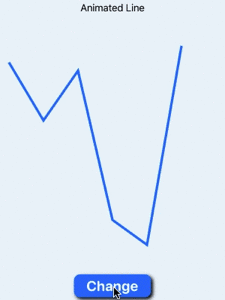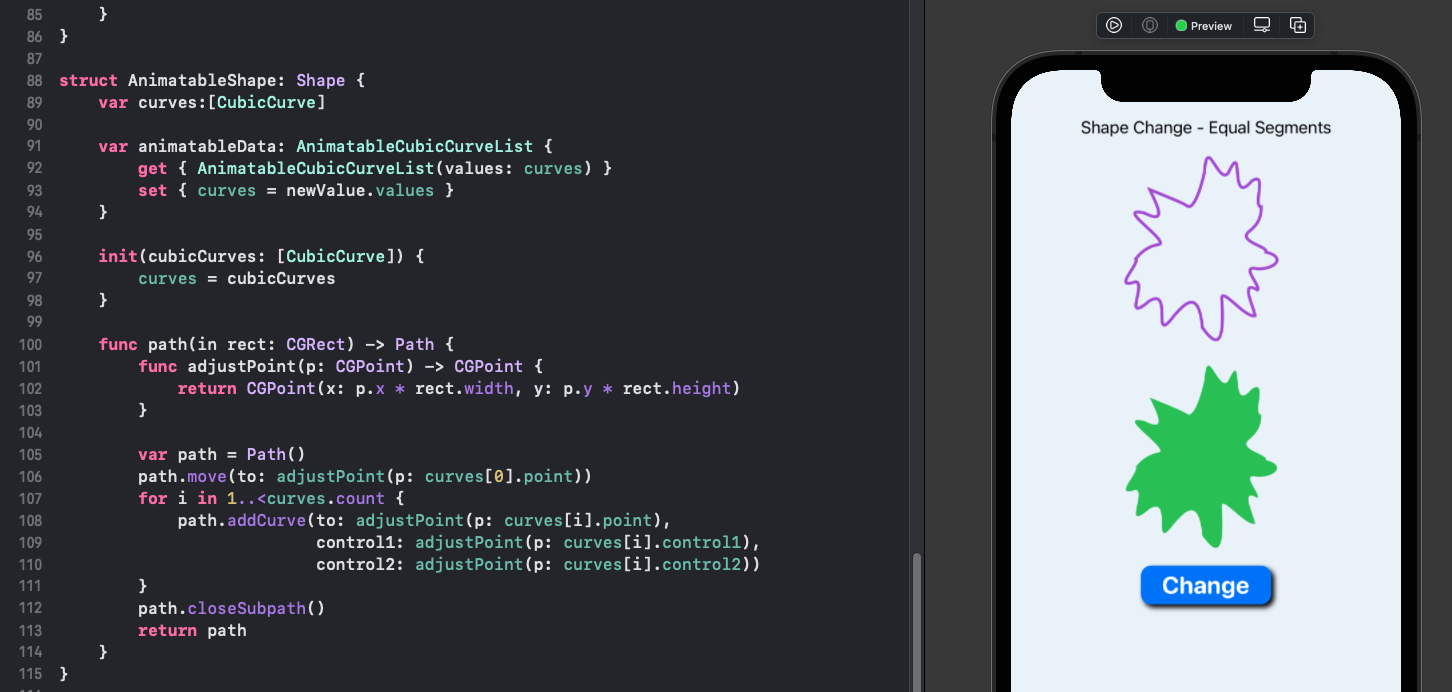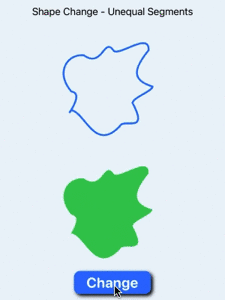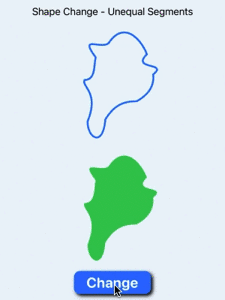# How to animate a Shape change in SwiftUI

Building on previous articles on Path animation in SwiftUI, this article demonstrates how to animate the smooth transition form one random shape to another. This is done by defining a custom AnimatableData for a list of cubic curves and using this in a custom shape so that SwiftUI can present a smooth transition from one shape to another.

This is the sixth article in a series on SwiftUI animation:

## Creating a random shape

A structure is defined for a CubicSegment that holds the end point and two control points to create the cubic Bézier curve. The function CreateRandomShape takes in the number of sides or segments in the shape and creates that number of random cubic Bézier curves around a central point in a 1 by 1 frame.

 1struct CubicSegment {
2    let point: CGPoint
3    let control1: CGPoint
4    let control2: CGPoint
5}
6
7func Cartesian(length:Double, angle:Double) -> CGPoint {
8    return CGPoint(x: length * cos(angle), y: length * sin(angle))
9}
10
11func CreateRandomShape(sides:Int) -> [CubicSegment] {
12    var segments:[CubicSegment] = []
13
14    let r = 0.5
15    let c = CGPoint(x: 0.5, y: 0.5)
16    let sectorAngle = 2.0 * Double.pi / Double(sides)
17    var previousSectorIn = true
18    for i in 0..<sides {
19        let segmentAngle = sectorAngle * Double.random(in: 0.7...1.3)
20        let angle = (sectorAngle * Double(i-0)) + segmentAngle
21        let radius = r * Double.random(in: 0.45...0.85)
22        let pt = Cartesian(length: radius, angle: angle)
23
24        let ctlAngle1 = angle - (segmentAngle * 0.75)
26        let ctl1 = Cartesian(length: ctlDistance1, angle: ctlAngle1)
27
28        let ctlAngle2 = angle - (segmentAngle * 0.25)
29        let ctlDistance2 = radius * Double.random(in: 0.55...1.45)
30        previousSectorIn = ctlDistance2 < radius
31        let ctl2 = Cartesian(length: ctlDistance2, angle: ctlAngle2)
32
33        let s:CubicSegment = CubicSegment(
34            point: CGPoint(x: pt.x + c.x, y: pt.y + c.y),
35            control1: CGPoint(x: ctl1.x + c.x, y: ctl1.y + c.y),
36            control2: CGPoint(x: ctl2.x + c.x, y: ctl2.y + c.y))
37        segments.append(s)
38    }
39    segments.append(segments)
40    return segments
41}


A Random shape is created from the array of CubicSegments with the size adjusted to fit into the containing frame. This conforms to the Shape protocol.

 1struct RandomShape: Shape {
2    var segments:[CubicSegment]
3
4    func path(in rect: CGRect) -> Path {
5        func adjustPoint(p: CGPoint) -> CGPoint {
6            return CGPoint(x: p.x * rect.width, y: p.y * rect.height)
7        }
8
9        var path = Path()
11        for i in 1..<segments.count {
15        }
16        path.closeSubpath()
17        return path
18    }
19}


Two variables of RandomShape are created and these shapes are swapped when the "Change" button is selected. Some attributes are animated such as color, but the actual shape change is not animated. This is because the SwiftUI framework does not know how to animate the transition from one list of CubicSegment to another.

 1struct ChangeShapeView: View {
2    @State private var changeShape = true
3
4    let segmentsShape1 = CreateRandomShape(sides: 5)
5    let segmentsShape2 = CreateRandomShape(sides: 5)
6
7    var body: some View {
8        VStack {
9            RandomShape(segments: changeShape ? segmentsShape1 : segmentsShape2)
10                .stroke(changeShape ? Color.purple : .red, lineWidth: 6.0)
11                .frame(width: 200, height: 200)
12                .animation(.easeInOut(duration: 2))
13
14            RandomShape(segments: changeShape ? segmentsShape1 : segmentsShape2)
15                .fill(changeShape ? Color.purple : .red)
16                .frame(width: 200, height: 200)
17                .animation(.linear(duration: 2))
18
19            Button("Change") {
20                self.changeShape.toggle()
21            }
22            .buttonStyle(BlueButtonStyle())
23
24            Spacer()
25        }
26    }
27}


Random shape created with array of cubic curves

## Use array of Animatable Cubic Curve

An AnimatableCubicCurve was defined in How to animate a Cubic Curve change in SwiftUI and these were combined in a view in a ZStack to create a shape. Each of these curves is Animatable, so could an array of these curves be used to define a shape and allow animation of a shape change? The short answer is no, because even if the individual segments are animatable, the shape of an array is not animatable. In order to animate a shape change, the SwiftUI framework needs to be able to calculate intermediate stage in the transition.

 1struct AnimatableCubicCurve : VectorArithmetic {
2    var start: CGPoint
3    var end: CGPoint
4    var control1: CGPoint
5    var control2: CGPoint
6
7    var length: Double {
8        return Double(((end.x - start.x) * (end.x - start.x)) +
9                        ((end.y - start.y) * (end.y - start.y))).squareRoot()
10    }
11
12    var magnitudeSquared: Double {
13        return length * length
14    }
15
16    mutating func scale(by rhs: Double) {
17        self.start.x.scale(by: rhs)
18        self.start.y.scale(by: rhs)
19        self.end.x.scale(by: rhs)
20        self.end.y.scale(by: rhs)
21        self.control1.x.scale(by: rhs)
22        self.control1.y.scale(by: rhs)
23        self.control2.x.scale(by: rhs)
24        self.control2.y.scale(by: rhs)
25    }
26
27    static var zero: AnimatableCubicCurve {
28        return AnimatableCubicCurve(start: CGPoint(x: 0.0, y: 0.0),
29                                    end: CGPoint(x: 0.0, y: 0.0),
30                                    control1: CGPoint(x: 0.0, y: 0.0),
31                                    control2: CGPoint(x: 0.0, y: 0.0))
32    }
33
34    static func - (lhs: AnimatableCubicCurve, rhs: AnimatableCubicCurve) -> AnimatableCubicCurve {
35        return AnimatableCubicCurve(
36            start: CGPoint(
37                x: lhs.start.x - rhs.start.x,
38                y: lhs.start.y - rhs.start.y),
39            end: CGPoint(
40                x: lhs.end.x - rhs.end.x,
41                y: lhs.end.y - rhs.end.y),
42            control1: CGPoint(
43                x: lhs.control1.x - rhs.control1.x,
44                y: lhs.control1.y - rhs.control1.y),
45            control2: CGPoint(
46                x: lhs.control2.x - rhs.control2.x,
47                y: lhs.control2.y - rhs.control2.y))
48    }
49
50    static func -= (lhs: inout AnimatableCubicCurve, rhs: AnimatableCubicCurve) {
51        lhs = lhs - rhs
52    }
53
54    static func + (lhs: AnimatableCubicCurve, rhs: AnimatableCubicCurve) -> AnimatableCubicCurve {
55        return AnimatableCubicCurve(
56            start: CGPoint(
57                x: lhs.start.x + rhs.start.x,
58                y: lhs.start.y + rhs.start.y),
59            end: CGPoint(
60                x: lhs.end.x + rhs.end.x,
61                y: lhs.end.y + rhs.end.y),
62            control1: CGPoint(
63                x: lhs.control1.x + rhs.control1.x,
64                y: lhs.control1.y + rhs.control1.y),
65            control2: CGPoint(
66                x: lhs.control2.x + rhs.control2.x,
67                y: lhs.control2.y + rhs.control2.y))
68    }
69
70    static func += (lhs: inout AnimatableCubicCurve, rhs: AnimatableCubicCurve) {
71        lhs = lhs + rhs
72    }
73
74    static func * (lhs: AnimatableCubicCurve, rhs: Double) -> AnimatableCubicCurve {
75        return AnimatableCubicCurve(
76            start: CGPoint(
77                x: lhs.start.x * CGFloat(rhs),
78                y: lhs.start.y * CGFloat(rhs)),
79            end: CGPoint(
80                x: lhs.end.x * CGFloat(rhs),
81                y: lhs.end.y * CGFloat(rhs)),
82            control1: CGPoint(
83                x: lhs.control1.x * CGFloat(rhs),
84                y: lhs.control1.y * CGFloat(rhs)),
85            control2: CGPoint(
86                x: lhs.control2.x * CGFloat(rhs),
87                y: lhs.control2.y * CGFloat(rhs)))
88    }
89}

 1struct CubicCurveShape: Shape {
2    var startPoint: CGPoint
3    var endPoint: CGPoint
4    var controlPoint1: CGPoint
5    var controlPoint2: CGPoint
6
7    private var animatableSegment: AnimatableCubicCurve
8
9    var animatableData: AnimatableCubicCurve {
10        get { AnimatableCubicCurve(
11            start: startPoint, end: endPoint, control1: controlPoint1, control2: controlPoint2) }
12        set {
13            startPoint = newValue.start
14            endPoint = newValue.end
15            controlPoint1 = newValue.control1
16            controlPoint2 = newValue.control2
17        }
18    }
19
20    init(startPoint: CGPoint, endPoint: CGPoint, controlPoint1: CGPoint, controlPoint2: CGPoint) {
21        self.startPoint = startPoint
22        self.endPoint = endPoint
23        self.controlPoint1 = controlPoint1
24        self.controlPoint2 = controlPoint2
25        self.animatableSegment = AnimatableCubicCurve(
26            start: startPoint,
27            end: endPoint,
28            control1: controlPoint1,
29            control2: controlPoint2)
30    }
31
32    init(curve: AnimatableCubicCurve) {
33        self.init(startPoint: curve.start,
34                  endPoint: curve.end,
35                  controlPoint1: curve.control1,
36                  controlPoint2: curve.control2)
37    }
38
39    func path(in rect: CGRect) -> Path {
40        let start = CGPoint(x: startPoint.x * rect.width,
41                            y: startPoint.y * rect.height)
42        let end = CGPoint(x: rect.width * endPoint.x,
43                          y: rect.height * endPoint.y)
44        let control1 = CGPoint(x: rect.width * controlPoint1.x,
45                               y: rect.height * controlPoint1.y)
46        let control2 = CGPoint(x: rect.width * controlPoint2.x,
47                               y: rect.height * controlPoint2.y)
48
49        var path = Path()
50        path.move(to: start)
51        path.addCurve(to: end, control1: control1, control2: control2)
52        return path
53    }
54}

 1func CreateRandomShape2(segments:Int) -> [AnimatableCubicCurve] {
2    var curves:[AnimatableCubicCurve] = []
3
4    let r = 0.5
5    let c = CGPoint(x: 0.5, y: 0.5)
6    let sectorAngle = 2.0 * Double.pi / Double(segments)
7    var previousSectorIn = true
8    var previousPoint = CGPoint(x: 0.0, y: 0.0)
9    for i in 0..<segments {
10        let segmentAngle = sectorAngle * Double.random(in: 0.7...1.3)
11        let angle = (sectorAngle * Double(i-0)) + segmentAngle
12        let radius = r * Double.random(in: 0.45...0.85)
13        let pt = Cartesian(length: radius, angle: angle)
14
15        let ctlAngle1 = angle - (segmentAngle * 0.75)
17        let ctl1 = Cartesian(length: ctlDistance1, angle: ctlAngle1)
18
19        let ctlAngle2 = angle - (segmentAngle * 0.25)
20        let ctlDistance2 = radius * Double.random(in: 0.55...1.45)
21        previousSectorIn = ctlDistance2 < radius
22        let ctl2 = Cartesian(length: ctlDistance2, angle: ctlAngle2)
23
24        let curve: AnimatableCubicCurve = AnimatableCubicCurve (
25            start: previousPoint,
26            end: CGPoint(x: pt.x + c.x, y: pt.y + c.y),
27            control1: CGPoint(x: ctl1.x + c.x, y: ctl1.y + c.y),
28            control2: CGPoint(x: ctl2.x + c.x, y: ctl2.y + c.y))
29        curves.append(curve)
30        previousPoint = curve.end
31    }
32    curves.append(curves)
33    return curves
34}

 1struct ShapeWithCubicCurveView: View {
2    @State private var changeShape = true
3
4    let segmentsShape1 = CreateRandomShape2(segments: 8)
5    let segmentsShape2 = CreateRandomShape2(segments: 8)
6
7    var body: some View {
8        VStack {
9            RandomShape2(segments: changeShape ? segmentsShape1 : segmentsShape2)
10                .stroke(changeShape ? Color.purple : .red, lineWidth: 6.0)
11                .frame(width: 200, height: 200)
12                .animation(.easeInOut(duration: 2))
13
14            RandomShape2(segments: changeShape ? segmentsShape1 : segmentsShape2)
15                .fill(changeShape ? Color.purple : .red)
16                .frame(width: 200, height: 200)
17                .animation(.linear(duration: 2))
18
19            Button("Change") {
20                self.changeShape.toggle()
21            }
22            .buttonStyle(BlueButtonStyle())
23
24            Spacer()
25        }
26    }
27}


Random shape created with array of animatable curves

## Animate an array of values

Previous shapes were animated by implementing Animatable protocol and supplying the AnimatableData. The AnimatableData has either been a single value or a number of values representing a single point or curve. Now we want to animate a series of values and the number of values in the series is open ended.

First animate a line that is composed of a list of doubles for it's "y" coordinates, while the "x" coordinates are distributed horizontally across the containing frame. The AnimatableLine structure is defined to contain the array of doubles and to conform to VectorArithmetic protocol. This is used as the AnimatableData for the LineShape shape.

This shows that it is possible to animate the transition from one set of values to another once the AnimatableData structure conforms to VectorArithmetic. This code will only work well with arrays that have the same number of samples. We'll deal with arrays of unequal length later when we deal with arrays of cubic curves.

 1struct AnimatableLine : VectorArithmetic {
2    var values:[Double]
3
4    var magnitudeSquared: Double {
5        return values.map{ $0 *$0 }.reduce(0, +)
6    }
7
8    mutating func scale(by rhs: Double) {
9        values = values.map{ 0 * rhs } 10 } 11 12 static var zero: AnimatableLine { 13 return AnimatableLine(values: [0.0]) 14 } 15 16 static func - (lhs: AnimatableLine, rhs: AnimatableLine) -> AnimatableLine { 17 return AnimatableLine(values: zip(lhs.values, rhs.values).map(-)) 18 } 19 20 static func -= (lhs: inout AnimatableLine, rhs: AnimatableLine) { 21 lhs = lhs - rhs 22 } 23 24 static func + (lhs: AnimatableLine, rhs: AnimatableLine) -> AnimatableLine { 25 return AnimatableLine(values: zip(lhs.values, rhs.values).map(+)) 26 } 27 28 static func += (lhs: inout AnimatableLine, rhs: AnimatableLine) { 29 lhs = lhs + rhs 30 } 31}   1struct LineShape: Shape { 2 var yValues: [Double] 3 4 var animatableData: AnimatableLine { 5 get { AnimatableLine(values: yValues) } 6 set { yValues = newValue.values } 7 } 8 9 func path(in rect: CGRect) -> Path { 10 let xIncrement = (rect.width / CGFloat(yValues.count)) 11 var path = Path() 12 path.move(to: CGPoint(x: 0.0, y: yValues * Double(rect.width))) 13 for i in 1..<yValues.count { 14 let pt = CGPoint(x: (Double(i) * Double(xIncrement)), y: (yValues[i] * Double(rect.height))) 15 path.addLine(to: pt) 16 } 17 return path 18 } 19}   1struct LineView: View { 2 @State private var changeShape = true 3 4 let line1 = [0.2, 0.4, 0.2, 0.8, 0.9, 0.1] 5 let line2 = [0.3, 0.9, 0.9, 0.8, 0.2, 0.5] 6 7 var body: some View { 8 VStack { 9 Text("Animated Line") 10 11 LineShape(yValues: changeShape ? line1 : line2) 12 .stroke(changeShape ? Color.blue : .red, lineWidth: 4.0) 13 .frame(width: 350, height: 420) 14 .animation(.easeInOut(duration: 2)) 15 16 Button("Change") { 17 self.changeShape.toggle() 18 } 19 .buttonStyle(BlueButtonStyle()) 20 21 Spacer() 22 } 23 } 24}Animate line change with an array of doubles Animate line change with an array of doubles ## Animate between shapes with the same number of sections We started with a list of doubles for an animatable line to demonstrate specifying AnimatableData with a list of values. We can build on this by first defining a struct CubicCurve to contain the three points required for a Cubic Bézier curve that conforms to VectorArithmetic.  1struct CubicCurve : VectorArithmetic { 2 var point: CGPoint 3 var control1: CGPoint 4 var control2: CGPoint 5 6 var magnitudeSquared: Double { 7 return 0.0 8 } 9 10 mutating func scale(by rhs: Double) { 11 self.point.x.scale(by: rhs) 12 self.point.y.scale(by: rhs) 13 self.control1.x.scale(by: rhs) 14 self.control1.y.scale(by: rhs) 15 self.control2.x.scale(by: rhs) 16 self.control2.y.scale(by: rhs) 17 } 18 19 static var zero: CubicCurve { 20 return CubicCurve(point: CGPoint(x: 0.0, y: 0.0), 21 control1: CGPoint(x: 0.0, y: 0.0), 22 control2: CGPoint(x: 0.0, y: 0.0)) 23 } 24 25 static func - (lhs: CubicCurve, rhs: CubicCurve) -> CubicCurve { 26 return CubicCurve( 27 point: CGPoint( 28 x: lhs.point.x - rhs.point.x, 29 y: lhs.point.y - rhs.point.y), 30 control1: CGPoint( 31 x: lhs.control1.x - rhs.control1.x, 32 y: lhs.control1.y - rhs.control1.y), 33 control2: CGPoint( 34 x: lhs.control2.x - rhs.control2.x, 35 y: lhs.control2.y - rhs.control2.y)) 36 } 37 38 static func -= (lhs: inout CubicCurve, rhs: CubicCurve) { 39 lhs = lhs - rhs 40 } 41 42 static func + (lhs: CubicCurve, rhs: CubicCurve) -> CubicCurve { 43 return CubicCurve( 44 point: CGPoint( 45 x: lhs.point.x + rhs.point.x, 46 y: lhs.point.y + rhs.point.y), 47 control1: CGPoint( 48 x: lhs.control1.x + rhs.control1.x, 49 y: lhs.control1.y + rhs.control1.y), 50 control2: CGPoint( 51 x: lhs.control2.x + rhs.control2.x, 52 y: lhs.control2.y + rhs.control2.y)) 53 } 54 55 static func += (lhs: inout CubicCurve, rhs: CubicCurve) { 56 lhs = lhs + rhs 57 } 58 59 static func * (lhs: CubicCurve, rhs: Double) -> CubicCurve { 60 return CubicCurve( 61 point: CGPoint( 62 x: lhs.point.x * CGFloat(rhs), 63 y: lhs.point.y * CGFloat(rhs)), 64 control1: CGPoint( 65 x: lhs.control1.x * CGFloat(rhs), 66 y: lhs.control1.y * CGFloat(rhs)), 67 control2: CGPoint( 68 x: lhs.control2.x * CGFloat(rhs), 69 y: lhs.control2.y * CGFloat(rhs))) 70 } 71}  Next define a struct AnimatableCubicCurveList that contains a list of these CubicCurve and also conforms to VectorArithmetic. We ignore dot product here and return zero for magnitudeSquared. In vector math, doProduct is commonly used to determine how aligned two vectors are and this is not appropriate for a list of cubic curves. This does not appear to be used for basic shape animation.  1struct AnimatableCubicCurveList : VectorArithmetic { 2 var values:[CubicCurve] 3 4 var magnitudeSquared: Double { 5 // dotProduct has no meaning on a list of cubic curves 6 return 0.0 7 } 8 9 mutating func scale(by rhs: Double) { 10 values = values.map {0 * rhs }
11    }
12
13    static var zero: AnimatableCubicCurveList {
14        return AnimatableCubicCurveList(values: [CubicCurve.zero])
15    }
16
17    static func - (lhs: AnimatableCubicCurveList, rhs: AnimatableCubicCurveList) ->
18    AnimatableCubicCurveList {
19        let result = zip(lhs.values, rhs.values).map(-)
20        return AnimatableCubicCurveList(values: result)
21    }
22
23    static func -= (lhs: inout AnimatableCubicCurveList, rhs: AnimatableCubicCurveList) {
24        lhs = lhs - rhs
25    }
26
27    static func + (lhs: AnimatableCubicCurveList, rhs: AnimatableCubicCurveList) ->
28    AnimatableCubicCurveList {
29        let result = zip(lhs.values, rhs.values).map(+)
30        return AnimatableCubicCurveList(values: result)
31    }
32
33    static func += (lhs: inout AnimatableCubicCurveList, rhs: AnimatableCubicCurveList) {
34        lhs = lhs + rhs
35    }
36}

 1struct AnimatableShape: Shape {
2    var curves:[CubicCurve]
3
4    var animatableData: AnimatableCubicCurveList {
5        get { AnimatableCubicCurveList(values: curves) }
6        set { curves = newValue.values }
7    }
8
9    func path(in rect: CGRect) -> Path {
10        func adjustPoint(p: CGPoint) -> CGPoint {
11            return CGPoint(x: p.x * rect.width, y: p.y * rect.height)
12        }
13
14        var path = Path()
16        for i in 1..<curves.count {
20        }
21        path.closeSubpath()
22        return path
23    }
24}


A helper function is defined to generate a random shape consisting of an array of CubicCurves. Note that the first point is appended to the end of the array as the point in this curve is first used to move the path to this point. This same point at the end of the path is used to define the cubic curve back to the starting point.

 1func CreateRandomShape3(segments:Int) -> [CubicCurve] {
2    var curves:[CubicCurve] = []
3
4    let r = 0.5
5    let c = CGPoint(x: 0.5, y: 0.5)
6    let sectorAngle = 2.0 * Double.pi / Double(segments)
7    var previousSectorIn = true
8    for i in 0..<segments {
9        let segmentAngle = sectorAngle * Double.random(in: 0.7...1.3)
10        let angle = (sectorAngle * Double(i-0)) + segmentAngle
11        let radius = r * Double.random(in: 0.45...0.85)
12        let pt = Cartesian(length: radius, angle: angle)
13
14        let ctlAngle1 = angle - (segmentAngle * 0.75)
16        let ctl1 = Cartesian(length: ctlDistance1, angle: ctlAngle1)
17
18        let ctlAngle2 = angle - (segmentAngle * 0.25)
19        let ctlDistance2 = radius * Double.random(in: 0.55...1.45)
20        previousSectorIn = ctlDistance2 < radius
21        let ctl2 = Cartesian(length: ctlDistance2, angle: ctlAngle2)
22
23        let curve = CubicCurve (
24            point: CGPoint(x: pt.x + c.x, y: pt.y + c.y),
25            control1: CGPoint(x: ctl1.x + c.x, y: ctl1.y + c.y),
26            control2: CGPoint(x: ctl2.x + c.x, y: ctl2.y + c.y))
27        curves.append(curve)
28    }
29    curves.append(curves)
30
31    return curves
32}


This shape now demonstrates a smooth animation from one shape to another.

 1struct AnimatableShapeView: View {
2    @State private var changeShape = true
3
4    let shape1 = CreateRandomShape3(segments: 19)
5    let shape2 = CreateRandomShape3(segments: 19)
6
7    var body: some View {
8        VStack {
9            Text("Shape Change - Equal Segments")
10
11            AnimatableShape(curves: changeShape ? shape1 : shape2)
12                .stroke(changeShape ? Color.purple : .red, lineWidth: 3.0)
13                .frame(width: 200, height: 200)
14                .animation(.easeInOut(duration: 2))
15
16            AnimatableShape(curves: changeShape ? shape1 : shape2)
17                .fill(changeShape ? Color.green : .yellow)
18                .frame(width: 200, height: 200)
19                .animation(.easeInOut(duration: 2))
20
21            Button("Change") {
22                self.changeShape.toggle()
23            }
24            .buttonStyle(BlueButtonStyle())
25
26            Spacer()
27        }
28    }
29}AnimatableShape that conforms to shape protocol and implements animatableData AnimatableShape that conforms to shape protocol and implements animatableData

Animate shape change when shapes have equal number of segments

## Animate between shapes of different number of segments

The above AnimatableShape works well when the number of Cubic Curves in the shapes to change are the same. However, when there is a difference in the number of curves the animation only works where the number of curves overlap. When switching from the larger one to the smaller one, the extra curves are first removed and then the remaining part of the shape is animated. Similarly, when transitioning to the larger one, the smaller shape is first animated and then the extra section just appears.

 1struct UnequalSegmentsView: View {
2    @State private var changeShape = true
3
4    let shape1 = CreateRandomShape3(segments: 8)
5    let shape2 = CreateRandomShape3(segments: 12)
6
7    var body: some View {
8        VStack {
9            Text("Shape Change - Unequal Segments")
10
11            AnimatableShape(curves: changeShape ? shape1 : shape2)
12                .stroke(changeShape ? Color.blue : .red, lineWidth: 3.0)
13                .frame(width: 200, height: 200)
14                .animation(.easeInOut(duration: 2))
15
16            AnimatableShape(curves: changeShape ? shape1 : shape2)
17                .fill(changeShape ? Color.green : .yellow)
18                .frame(width: 200, height: 200)
19                .animation(.easeInOut(duration: 2))
20
21            Button("Change") {
22                self.changeShape.toggle()
23            }
24            .buttonStyle(BlueButtonStyle())
25
26            Spacer()
27        }
28    }
29}Jumpy animation of shape change when shapes have unequal numbers of segments
Animate shape change when shapes have unequal number of segments

## Improving animation of shape with different number of segments

We need to change the + and - functions on the AnimatableCubicCurveList to address the smooth animation between shapes with different numbers of curves. Essentially, we need to add curves to the shorter list so that the two shapes contain the same number of curves. Two helper functions are added in AnimatableCubicCurveList2; equalizeArrays is a function to ensure the two arrays are of equal size and this is used in both the + and - functions; padShorterList functions is called by equalizeArrays and creates a curve matching all three points in the curve with the first point of the shorter array and adding repeats of this to the end of the array until the sizes match. This will have the effect of collapsing all the extra curves in the larger shape onto the first/last point of the smaller shape during the transition. The new curves for the larger shape will animate from the start point of the smaller shape.

 1struct AnimatableCubicCurveList2 : VectorArithmetic {
2    var values:[CubicCurve]
3
4    var magnitudeSquared: Double {
5        // dotProduct has no meaning on a list of cubic curves
6        return 0.0
7    }
8
9    mutating func scale(by rhs: Double) {
10        values = values.map { \$0 * rhs }
11    }
12
13    static var zero: AnimatableCubicCurveList2 {
14        return AnimatableCubicCurveList2(values: [CubicCurve.zero])
15    }
16
17    fileprivate static func padShorterList(_ shortList: inout [CubicCurve], _ longList: [CubicCurve]) {
18        // Create a curve with all points set to the first/last point
19        // Extend the shorter list with repeats of this point
20        let point = shortList.point
21        let curve = CubicCurve(point: point, control1: point, control2: point)
22        for _ in(shortList.count..<longList.count) {
23            shortList.append(curve)
24        }
25    }
26
27    fileprivate static func equalizeArrays(_ lhs: AnimatableCubicCurveList2, _ rhs: AnimatableCubicCurveList2) -> ([CubicCurve], [CubicCurve]) {
28        var leftValues = lhs.values
29        var rightValues = rhs.values
30        if leftValues.count < rightValues.count {
32        }
33        else if rightValues.count < leftValues.count {
35        }
36        return (leftValues, rightValues)
37    }
38
39    static func - (lhs: AnimatableCubicCurveList2, rhs: AnimatableCubicCurveList2) -> AnimatableCubicCurveList2 {
40        // Ensure the shapes have the same number of curves
41        let lists = equalizeArrays(lhs, rhs)
42        let result = zip(lists.0, lists.1).map(-)
43        return AnimatableCubicCurveList2(values: result)
44    }
45
46    static func -= (lhs: inout AnimatableCubicCurveList2, rhs: AnimatableCubicCurveList2) {
47        lhs = lhs - rhs
48    }
49
50    static func + (lhs: AnimatableCubicCurveList2, rhs: AnimatableCubicCurveList2) -> AnimatableCubicCurveList2 {
51        // Ensure the shapes have the same number of curves
52        let lists = equalizeArrays(lhs, rhs)
53        let result = zip(lists.0, lists.1).map(+)
54        return AnimatableCubicCurveList2(values: result)
55    }
56
57    static func += (lhs: inout AnimatableCubicCurveList2, rhs: AnimatableCubicCurveList2) {
58        lhs = lhs + rhs
59    }
60}

 1struct AnimatableShape2: Shape {
2    var curves:[CubicCurve]
3
4    var animatableData: AnimatableCubicCurveList3 {
5        get { AnimatableCubicCurveList3(values: curves) }
6        set { curves = newValue.values }
7    }
8
9    func path(in rect: CGRect) -> Path {
10        func adjustPoint(p: CGPoint) -> CGPoint {
11            return CGPoint(x: p.x * rect.width, y: p.y * rect.height)
12        }
13
14        var path = Path()
16        for i in 1..<curves.count {
20        }
21        path.closeSubpath()
22        return path
23    }
24}Smooth animation of shape change when shapes have unequal numbers of segments
Smooth animation of shape change when shapes have unequal numbers of segments

In a previous article, an array of AnimatableCubicCurve were arranged in a ZStack to present a shape. This provided a good outline of a shape, but cannot be used as a shape, such as the fill modifier cannot be applied. The AnimatableShape2 defined in this article defines a random shape composed of a number of cubic Bézier curves and is a true shape. It allows for smooth animation between different random shapes as well as scaling and color change.